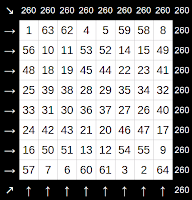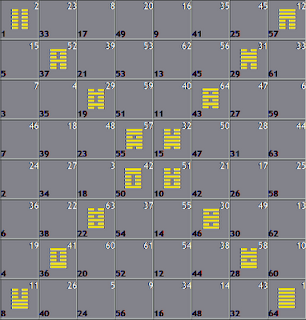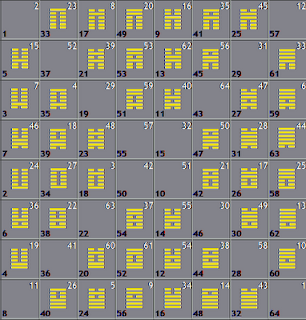## Sunday, March 3, 2013

### Fu Xi and King Wen

Fu Xi 's complementary arrangement and King Wen's pairs coincide on the 8 x 8 square

Wen's pairs can be said to fall into two categories: 8 pairs that are complementary, and 24 pairs that are figurate inverses.

The pairs of the first kind are found along both of the major diagonals which reflect across each other.  The pairs of the second kind are found reflected across the vertical axis of the diagram.

The colors indicate the line-composition of the figures:
Black: 6 YANG 0 YIN
Indigo: 5 YANG 1 YIN
Blue: 4 YANG 2 YIN
Green: 3 YANG 3 YIN
Yellow: 2 YANG 4 YIN
Orange: 1 YANG 5 YIN
Red: 0 YANG 6 YIN

### Fu Xi's arrangement, Wen's pairs, and the 8 x 8 square

This diagram shows the affinity between Wen's pairing and Fu Xi's arrangement of complementary opposites on the 8 x 8 square.

## Wednesday, January 16, 2013

### "Heaven and Earth, that is Tao"

In the first chapter of The Great Treatise we find:
The changes and transformations of Heaven are in the symbols of Change
The changes and transformations of Earth are in the forms of Change

Also:
What moves and completes the symbols is called Ch'ien.
What unfolds them into patterns of living is called K'un.

Thus, Ch'ien represents enfolded or implicate order, creative, symbolic reality; while K'un is explicated/unfolded, structive, formative reality.

Earth (K'un) is represented by the form of the simple square.
Heaven (Ch'ien) is immaterial or intangible and has no form as such, but we may represent it through an overlaid series of lines that partition the square into four discrete regions:

The resulting figure can be resolved into fractions of sixteen.  The respective areas of the four discrete regions are 1/16, 3/16, 5/16, and 7/16, comprising a unity.  These four fractions are identical to the probabilities, given by the yarrow oracle, of measuring the four xiang symbols during divination.

Having received the four symbols by combining Heaven and Earth, we project them onto our figure, yielding a full field to support the existence of the 64 Images:

From this we can infer that the  Eight Diagrams emerge from the 64 Images, consistent with Dr. Stephen Karcher's argument that the 64 Images "preceded" the Eight Diagrams (Ba Gua), which are used for identification and interpretation of the Images.

The ancients are said to have devised many implements by imitating Change.  Here, and elsewhere, I suggest that that the yarrow oracle was devised to imitate the intermingling of Heaven and Earth that reveals the xiang.  The figures presented here are purely geometric, hence they are antecedent of human artifice.  The xiang are eternal; coeval with, and fairly indistinct or inseparable from Change.  The ancient sages' far-reaching powers of sentience and cognition brought forth the xiang into consensus reality for the use of mankind.

The point that I hope to have made is that the xiang are entangled with Change, embedded or encoded in Change by way of this intermingling of the square (representing Earth) and the enfoldment that Heaven represents.  Without the enfoldment, we cannot discern the symbols in the square; without the square, the symbols have no basis for existence.  Indeed, "Heaven and Earth, that is Tao."

If we accept this line of reasoning, we can posit a natural, mathematical interpretation for Change that can be extended with further investigation.

## Tuesday, January 1, 2013

### Quantum Measurement

Systems exhibiting tangled hierarchy are said to have some degree of self-awareness or 'soul', per the writings of Douglas Hofstadter.  The following illustrations are provided to demonstrate the tangled hierarchy of the system called Change.

Physicist Amit Goswami argues that systems demonstrating tangled hierarchy (plus non-locality and discontinuity) are related to quantum systems.  A sub-theme of these illustrations concerns observers and measurement, salient themes of quantum mechanics

In the Field diagram above, the four concentric regions indicate four "densities" and represent the four Symbols.
The numbers in the diagram indicate the areas of the bounded regions enclosing those numbers.
Each quadrant of the diagram encloses an area of 16 units; thus the two cardinal axes and four densities enfold 6 dimensions and 64 Elements.

We never experience any of the 64 elements directly; we perceive them only indirectly by way of the four Symbols, which we can experience probabilistically, through random selection detailed by the measurement Ritual.

The four Symbols, represented on the Field with colors, give us probability densities represented by their respective bounded areas.  The densities reflect the chance of sampling the given Symbol during the measurement Ritual.

The Field comprises six dimensions.  An object represented on the Field, or by the Field, or which existence depends on the Field, should reasonably comprise 6 dimensions.  Likewise, any such object should be expressible in terms of 6 dimensions.
The figure above right is a stack made of six Fields; thus, these six Places are means of expressing the 6 dimensions.

The Ritual is conducted upon the Field (above left) by randomly sampling a single Symbol from each of its six dimensions, represented by the stack (above center), then interpreting the ordered collection of samples (above right) as one of the 64 Elements.

Questions:
What is the subject of measurement?
What is the object of measurement?

## Thursday, September 20, 2012

### Sphere: SquaredIn the diagram at right, each figure's cell is identified by two numbers: traditional sequence at upper-right, and scalar quantity at lower-left.
This 64-cell grid is arranged so that each cell is placed at 180 degrees of rotation from its logical complement.

Other ways of expressing this spatial relationship are that the complementary figures are maximally-separated, that they are antipodally-
positioned, or that they are diametrically-arranged .

Complementary hexagram pairs comprise a symbolic whole when their scalar values are summed, represented by the number 65.  Seen together, one figure will have YANG lines where the other figure has YIN lines.

In the diagram following, complementary relationships are also depicted by color pairs: RED with VIOLET, ORANGE with INDIGO, YELLOW with BLUE, or GREEN with GREEN.  Complementary pairs on the 8 x 8 grid are precisely analogous to antipodally-positioned hexagram pairs on any of the thirty-two spherical axes.

Key

RED + VIOLET = 2 polar cells (absolutely polarized)ORANGE + INDIGO = 12 cells (strongly polarized)
YELLOW + BLUE = 30 cells (weakly polarized)
GREEN = 20 equatorial cells (non-polarized)

Explication

RED and VIOLET figures establish a pole that vertically bisects the grid
YELLOW and BLUE figures are located close to GREEN and far from RED and VIOLET, thus they are weakly polarized: young, static, stable
ORANGE and INDIGO figures are located close to the RED and VIOLET polar figures, thus they are strongly polarized: old, dynamic, changing
GREEN figures horizontally bisect the grid, are located between BLUE and YELLOW, and are generally farthest from the poles; thus they are neutrally- or non-polarized

Classified per line-type count,

YELLOW and ORANGE have predominantly open lines and have YIN character
GREEN have YANG and YIN in equal measure
BLUE and INDIGO have predominantly closed lines and are YANG in character

NOTES:

Wen's traditional pairing schema is preserved within the xian tian arrangement.  Wen's pairs are defined as
1. a figure pairing with its figurate inversion where such inversion produces a figure different from the original; otherwise by
2. a figure mated to its logical negation, as in the case of (#01, #02).
Icosahedral characteristics
30 EDGES, 20 triangular FACES, 12 VERTICES

Dodecahedral characteristics
30 EDGES, 12 pentagonal FACES, 20 VERTICES

xiang::nucleotide bases: adenine + uracil  & cytosine + guanine
hexagram equivalent in RNA language: three nucleic acids :: codon :: hexagram

xiang::nucleotide pairing schema:

adenine: (INDIGO, old yang, 9) + uracil (ORANGE, old yin, 6)
cytosine (YELLOW, young yin, 8) + guanine (BLUE, young yang, 7)

ROYGBIV Color "octave"

Figures #01 and #02, located in the diagram at the high and low corners, are poles of the reference axis.  The remaining 62 paths, or 31 axes are "free"

Human peripheral nervous system comprises 31 nerve pairs

Tun calendar :: 18 * 20 = 360

Tzolkin calendar :: 13 * 20 = 260

20 * (13 + 18) = 20 * (31) = 620

## Wednesday, September 19, 2012

### Tun, Tzolkin, and XMS

The Maya are said to have used many calendars, several of which remain unknown to outsiders.  Three of their calendars are fairly-well known; these calendars are based on the standard 24-hour day, called kin.
• Tun (literally, stone) is regarded as a prophetic calendar by the Maya people.  It comprises 360 kin partitioned into 18 uinal (or winal, month), each with 20 kin.
• Tzolkin is the sacred calendar of the Maya; it comprises 20 periods of thirteen kin, or 260 days.  Together these two form the basis of the Maya timekeeping system.
• Haab (365 kin) reflected the Mayan solar year, and was used as a civil calendar, strictly used for accounting, taxation, and perhaps agriculture.
All Mayan calendar dates, including personal birthdays, dedications and most sacred ceremonial events were measured in 360-day periods called, tuns.  All dates "carved in stone" throughout the Mayan and Toltec world are tun dates...
This Tun, 360 day calendar is the only Maya calendar directly connected to the Tzolkin and they run together like two gears, each day being a tooth on the respective gears.
J. Eric Thompson's paper:
J. T. Goodman wrote of the 360-day period, now called the Tun: “This period is the real basis of the Maya chronological system.”
Furthermore, every known unit in the Maya calendar has in its composition the symbol for the 360-day year....
C. J. Calleman's statements:
The real point to get for the study of the Mayan calendar is however that in its prophetic uses it is based on the 260-day tzolkin or the 360-day tun, cycles that do not have an origin in the physical universe.18 "spells" of xiantian magic square
Xiantian Magic Square
The XMS arrangement comprises eight columns, eight rows, and two diagonals for a total of 18 groups linearly-arranged.  We can also refer to the groups as octets or "spells."  Each of the eighteen "spells" comprises a unique collection of eight numbers all arranged in a line on the grid.  Additionally, each octet sums to 260, the same number of days as in the tzolkin.
• Each octet may stand for a winal (20-day tun month) since both octets and uinals relate to  eighteen
• Since an octet represents 20 days (winal) and sums to 260 (tzolkin year), it  associates each day of an octet with the value 13
Considered this way, XMS can be considered as relating to both tun and tzolkin calendars, though our use of metaphor may somewhat exaggerate these relations.  It should be noted that the number 20 is not yet directly discernible within the XMS arrangement, though it is a demonstrable feature of the 64 hexagrams (q.v. yao-groups).  The equatorial plane of the spherical representation of Change comprises twenty complementary pairs.

Notes:
Complementary pairs on the XMS grid sum to 65; any four such pairs sum to 260, the same number of days in the tzolkin calendar.  Four pair is an octet, an octet is 20 days; therefore one pair is five days (value 65) and one day has value 13.  QED

Coincidentally, 13 / 8 * 360 (tun) = 585, a fair approximation to the Venus cycle of 584.  Admittedly, 8 / 5 * 365 (haab) = 584; a precise fit.  Both fractions (13/8 and 8/5) are common approximations to the golden ratio, phi (~1.618).

Scientists have calculated that approximately 1 billions of years ago, the Moon was ~25% closer than it is today, the Earth having an ~18-hour day and a 18 - 20-day month as marked by the Moon's circuit.

Linear octants are also called "spells" in acknowledgement of the 18 spells gained by Odin through his self-sacrifice on the World Tree Yggdrasil: two for each day he was hanged.

## Tuesday, September 18, 2012

### Axes and Reflections on the 8 x 816 axial figures
Ashtapada, the 8 x 8 field, is here populated with xian tian (complementary opposition) hexagram arrangement.  We observe that two pairs of orthogonal axes (opposed at 90 degrees) cross the field.  These axes include HORIZONTAL with VERTICAL, and DIAGONAL with SLANT.
The 48 remaining cells of the field are effectively partitioned into four groups of twelve contiguous cells by the SLANT and DIAGONAL axes.  The VERTICAL and HORIZONTAL axes further bisect these four groups of 12 cells.
• HORIZONTAL axis (unmarked) divides the field into an upper half and a lower half
• VERTICAL axis (unmarked) divides the field into left and right halves
• DIAGONAL axis is defined by the eight cells that span the corners lower left to upper right, or conversely.
• SLANT axis is defined by the eight cells that span the corners lower right to upper left, or conversely.
It should be noted that the figures located on the DIAGONAL and SLANT axes exhibit the associated relationships when they are folded or reflected across each other.  Example: figures (#52,#53) located on the SLANT axis (which crosses the DIAGONAL), are related through transposing and complementing both trigrams.48 non-axial figures

REFLECTIONS ACROSS AN AXIS
Imagine our grid printed on a square piece of paper which is then folded across length and width, and across both diagonals.  An axis is equivalent to any singular fold-line; reflection across an axis results in two cells that "mirror" each other on either side of the fold-line.  If the paper were actually folded as described, the mirrored cells would overlap perfectly.  Now with a working definition of axial reflection over our grid, let's look at relationships among the reflected figures.

1. Reflection across the HORIZONTAL axis complements a figure's lower trigram, leaving the upper trigram unchanged; e.g.: (#46,#24)**
2. Reflection across the VERTICAL axis complements a figure's  upper trigram, leaving the lower trigram unchanged; e.g.: (#59,#40)
3. Reflection across the SLANT axis transposes OR complements both trigrams of a figure; e.g.: (#28,#61)
4. Reflection across the DIAGONAL axis transposes AND complements both trigrams of a figure; e.g.: (#4,#38)  ** parenthesized numbers indicate the traditional hexagram ordering, located at upper-right corner of cells in white text.
Likewise, paired figures (#11,#12) located on the DIAGONAL axis (which crosses the SLANT), are related through transposing trigrams; alternatively, through complementing trigrams.
Reflection across a single axis is equivalent to rotating the grid in place by 90 degrees; therefore, reflection across two orthogonal axes is equivalent to rotating the grid in place by 180 degrees.

For any figure selected, crossing two orthogonal axes results in complementing the entire figure.  This fact is intrinsic to the xian tian arrangement whereby pairs of complementary hexagrams are separated on the field by 180 degrees of rotation, or two orthogonal axes.   Since DIAGONAL and SLANT axes cross both HORIZONTAL and DIAGONAL axes, the reflections of the figures on those axes are complementary.

No matter the combination of axial reflections, any selected figure and its several reflections remain confined within the same concentric band of figures on the field.  These are termed xiang probability bands because of their relation to the divinatory probabilities for generating each of the four kinds of lines (xiang) that may appear when consulting the oracle.

XMS (XIANTIAN MAGIC SQUAREelsewhere described in detail) is a magic square that is also a xian tian or complementary opposition arrangement; therefore, the relationships described above likely also hold for XMS.

This diagram embodies many of the same qualities as the 8 x 8 xiantian diagramxiang probability bands

NB: The two outer bands of the xiang probability square representing static YIN and static YANG contain 28 + 20 = 48 cells, the same number as the non-axial cells Therefore, these cells may stand for static YIN with static YANG, or a stable condition overall.  The same logic represents the axial cells as 12 + 4 = 16, dynamic YANG with dynamic YIN, a changing condition.